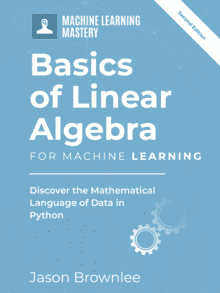# Linear Algebra Cheat Sheet for Machine Learning

Last Updated on October 17, 2021

### All of the Linear Algebra Operations that You Need to Use in NumPy for Machine Learning.

The Python numerical computation library called NumPy provides many linear algebra functions that may be useful as a machine learning practitioner.

In this tutorial, you will discover the key functions for working with vectors and matrices that you may find useful as a machine learning practitioner.

This is a cheat sheet and all examples are short and assume you are familiar with the operation being performed.

Kick-start your project with my new book Linear Algebra for Machine Learning, including step-by-step tutorials and the Python source code files for all examples.

Let’s get started.Linear Algebra Cheat Sheet for Machine Learning
Photo by Christoph Landers, some rights reserved.

## Overview

This tutorial is divided into 7 parts; they are:

1. Arrays
2. Vectors
3. Matrices
4. Types of Matrices
5. Matrix Operations
6. Matrix Factorization
7. Statistics

### Need help with Linear Algebra for Machine Learning?

Take my free 7-day email crash course now (with sample code).

Click to sign-up and also get a free PDF Ebook version of the course.

## 1. Arrays

There are many ways to create NumPy arrays.

## 2. Vectors

A vector is a list or column of scalars.

## 3. Matrices

A matrix is a two-dimensional array of scalars.

## 4. Types of Matrices

Different types of matrices are often used as elements in broader calculations.

## 5. Matrix Operations

Matrix operations are often used as elements in broader calculations.

## 6. Matrix Factorization

Matrix factorization, or matrix decomposition, breaks a matrix down into its constituent parts to make other operations simpler and more numerically stable.

## 7. Statistics

Statistics summarize the contents of vectors or matrices and are often used as components in broader operations.

### Linear Least Squares

This section provides more resources on the topic if you are looking to go deeper.

## Summary

In this tutorial, you discovered the key functions for linear algebra that you may find useful as a machine learning practitioner.

Are there other key linear algebra functions that you use or know of?
Let me know in the comments below.

Do you have any questions?

## Get a Handle on Linear Algebra for Machine Learning!#### Develop a working understand of linear algebra

...by writing lines of code in python

Discover how in my new Ebook:
Linear Algebra for Machine Learning

It provides self-study tutorials on topics like:
Vector Norms, Matrix Multiplication, Tensors, Eigendecomposition, SVD, PCA and much more...

### 13 Responses to Linear Algebra Cheat Sheet for Machine Learning

1.Azhaar February 23, 2018 at 5:22 am #

Very helpful! thanks for compiling and sharing this cheat sheet.

•Jason Brownlee February 23, 2018 at 12:03 pm #

I’m glad to hear that it helps.

2.Matthew February 23, 2018 at 1:09 pm #

Thanks Jason, it is very helpful. I like it!

•Jason Brownlee February 24, 2018 at 9:08 am #

Thanks Matthew!

•Gerardo October 28, 2021 at 12:17 pm #

Thank you for sharing! Good info inside

3.Balaji Venkateswaran February 23, 2018 at 4:08 pm #

Thanks for this quick cheat sheet. Very useful one!

•Jason Brownlee February 24, 2018 at 9:09 am #

Thanks.

4.Akhtar February 23, 2018 at 5:13 pm #

Thanks Jason, this is super helpful.

•Jason Brownlee February 24, 2018 at 9:10 am #

5.Damian May 4, 2018 at 10:32 pm #

Hello.
Thanks for this summary.
A small remark:
Matrix-Scalar Multiplication doesn’t work exclusively like that (at least in python 3.6)
you are able to use either A*scalar(k) or np.dot (k).
And it makes more sense to respect original math notations and not to abuse the function by using a scalar with it.

•Jason Brownlee May 5, 2018 at 6:19 am #

Thanks.

6.Donald Lacombe October 26, 2021 at 1:41 am #

One can save a few keystrokes by using the @ symbol for the dot product:

A@b

•Adrian Tam October 27, 2021 at 2:27 am #

Yes, you’re right. But only available in Python 3.5 and afterwards.